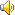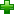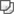# equivalent [i'kwivələnt]n. 等价物，相等物

# 词汇辨析

equal，equivalent
这两个形容词均有“相等的”之意
equal   指几个物或事在数目、数量、重量、程度或价值等方面完全相等。
equivalent   指几个物或事彼此对等，或者具有同等的价值、效果或意义等。

# 英英解释

n.
1. a person or thing equal to another in value or measure or force or effect or significance etc
2. the atomic weight of an element that has the same combining capacity as a given weight of another el
1. equal in amount or value
2. being essentially equal to something

# 网络释义

equivalent
等效量; 等效; 等价的; 相等的;
等效构件;
Equivalent equation
等价方程式; 同解方程，等价方程; 同解方程; 等价方程;
water equivalent
水当量; 水當量; 释义：水当量; 含水当量;
electrochemical equivalent
电化当量; 电流当量; 电化[学]当量; 电化当量=>电気化学当量;